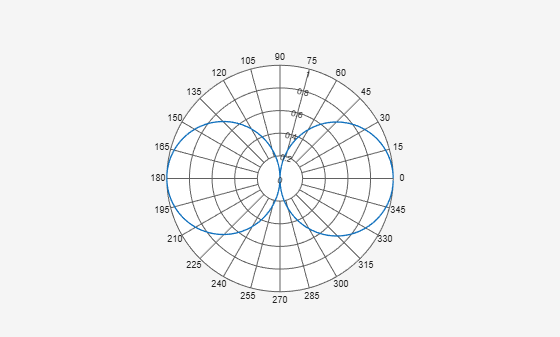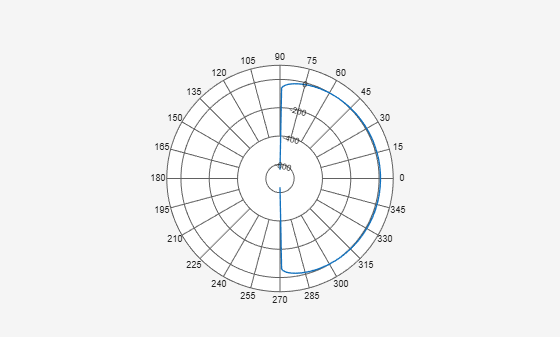Add data to polar plot

## Syntax

``add(p,d)``
``add(p,angle,magnitude)``

## Description

example

````add(p,d)` adds new antenna data to the polar plot, `p` based on the real amplitude values, `data`. ```

example

````add(p,angle,magnitude)` adds data sets of `angle` vectors and corresponding `magnitude` matrices to polar plot `p`.```

## Input Arguments

expand all

Polar plot, specified as a scalar handle.

Antenna or array data, specified as one of the following:

• A real length-M vector, where M contains the magnitude values with angles assumed to be $\frac{\left(0:M-1\right)}{M}×{360}^{\circ }$ degrees.

• A real M-by-N matrix, where M contains the magnitude values and N contains the independent data sets. Each column in the matrix has angles taken from the vector $\frac{\left(0:M-1\right)}{M}×{360}^{\circ }$ degrees. The set of each angle can vary for each column.

• A real N-D array, where N is the number of dimensions. Arrays with dimensions `2` and greater are independent data sets.

• A complex vector or matrix, where `data` contains Cartesian coordinates ((x,y) of each point. x contains the real part of `data` and y contains the imaginary part of `data`.

When data is in a logarithmic form such as dB, magnitude values can be negative. In this case,`polarpattern` plots the lowest magnitude values at the origin of the polar plot and highest magnitude values at the maximum radius.

Set of angles, specified as a vector in degrees.

Set of magnitude values, specified as a vector or a matrix. For a matrix of magnitude values, each column is an independent set of magnitude values and corresponds to the same set of angles.

## Examples

expand all

Create a cosine-pattern antenna and plot the pattern from 0° to 36°.

```az = [0:360]; p1 = abs(cosd(az));```

Plot the polar pattern.

`P = polarpattern(p1);`Create a second cosine-pattern antenna rotated by 60°. Add this pattern to the existing pattern.

```p2 = abs(cosd(az - 50)); add(P,p2);```Create a cosine antenna and plot the polar pattern of its directivity at 75 MHz.

```cosineantenna = phased.CosineAntennaElement('FrequencyRange',[1.0e0 100.0e9],... 'CosinePower',[2,2]); p1 = pattern(cosineantenna,75.0e6,[-90:90],0,'Type','Directivity'); P = polarpattern([-90:90],p1);```Create an isotropic antenna. Calculate the directivity of this antenna at 75 MHz.

```isoantenna = phased.IsotropicAntennaElement('FrequencyRange',... [1.0e0 100.0e9]); p2 = pattern(isoantenna,75.0e6,[-180:180],0,'Type','Directivity');```

Add the directivity plot of the isotropic antenna to the directivity plot of the cosine antenna.

`add(P,[-180:180],p2);`## Version History

Introduced in R2016a